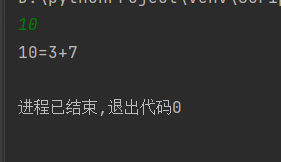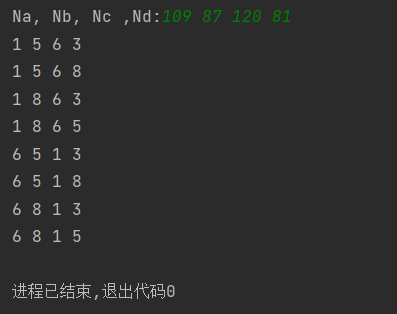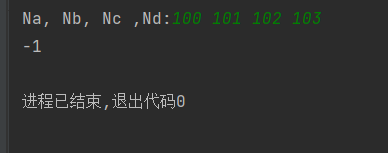## 程序员最近都爱上了这个网站  程序员们快来瞅瞅吧！  it98k网:it98k.com

出租广告位,需要合作请联系站长

+关注

14天阅读挑战赛

题目描述

# 算法题目描述

## 哥德巴赫猜想

10

10=3+7

### 代码实现

``def zhishu(num):    q = True    if num==1:            q = False    for i in range(2, num):        if num % i == 0:            q= False    return qdef guess(a,b,num):    if a<num and b>0:        if zhishu(a) == True and zhishu(b) == True:            print('%d=%d+%d' % (num,a,b))        else:            return guess(a+2,b-2,num)num = int(input())a = 1b=num-1guess(a,b,num)``

### 执行结果## 教室排课

### 题目描述

试编一个程序，为上述四个教学班分配教室。找出所有可行的分配方案，对于每个方案依次输出为专业A、B、C、D分配的教室编号，输出所有方案。
输入格式
一行，包含4个整数Na, Nb, Nc，Nd (20≤Na, Nb, Nc，Nd≤120)，每2个整数之间用一个空格隔开。

如果存在分配方案，输出若干行，每行表示一种教室分配方案，包含4个整数，依次表示A、B、C、D四个专业分配的教室编号。
如果不存在分配方案，输出-1。
样例输入：
109 87 120 81
样例输出：
1 5 6 3
1 5 6 8
1 8 6 3
1 8 6 5
6 5 1 3
6 5 1 8
6 8 1 3
6 8 1 5

100 101 102 103

-1

### 代码实现

``js=[120,40,85,50,100,140,70,100]flag=-1Na, Nb, Nc ,Nd= map(int, input('Na, Nb, Nc ,Nd:').split())for i in range(len(js)):    if Na>js[i]:        continue    for j in range(len(js)):        if(j==i or Nb>js[j]):            continue        for k in range(len(js)):            if(k==i or k==j or Nc>js[k]):                continue            for p in range(len(js)):                if(p==i  or p==j or p==k or Nd>js[p]):                    continue                print(i + 1,j+1,k+1,p+1)                flag=1if flag==-1:    print(flag)``

### 运行结果10 0

pdf(new) 更多>